1669281145

# Python Matplotlib Library with Examples

Matplotlib Tutorial – Python Matplotlib Library with Examples

In my previous blog, I discussed about a numerical library of python called Python NumPy. In this blog, I will be talking about another library, Python Matplotlib. matplotlib.pyplot is a python package used for 2D graphics. Learning to use this library efficiently is also an essential part of Python Certification curriculum. Below is the sequence in which I will be covering all the topics of python matplotlib:

• What Is Python Matplotlib?
• What is Matplotlib used for?
• Types Of Plots
–  Bar Graph
– Histogram
– Scatter Plot
– Area Plot
– Pie Chart
• Working With Multiple Plots

## What Is Python Matplotlib?

matplotlib.pyplot is a plotting library used for 2D graphics in python programming language. It can be used in python scripts, shell, web application servers and other graphical user interface toolkits.

## What is Matplotlib used for?

Matploitlib is a Python Library used for plotting, this python library provides and objected-oriented APIs for integrating plots into applications.

### Is Matplotlib Included in Python?

Matplotlib is not a part of the Standard Libraries which is installed by default when Python, there are several toolkits which are available that extend python matplotlib functionality. Some of them are separate downloads, others can be shipped with the matplotlib source code but have external dependencies.

• Basemap: It is a map plotting toolkit with various map projections, coastlines and political boundaries.
• Cartopy: It is a mapping library featuring object-oriented map projection definitions, and arbitrary point, line, polygon and image transformation capabilities.
• Excel tools: Matplotlib provides utilities for exchanging data with Microsoft Excel.
• Mplot3d: It is used for 3-D plots.
• Natgrid: It is an interface to the natgrid library for irregular gridding of the spaced data.

You may go through this recording of Python Matplotlib where our instructor has explained how to download Matplotlib in Python and the topics in a detailed manner with examples that will help you to understand this concept better.

## Python Matplotlib : Types of Plots

There are various plots which can be created using python matplotlib. Some of them are listed below: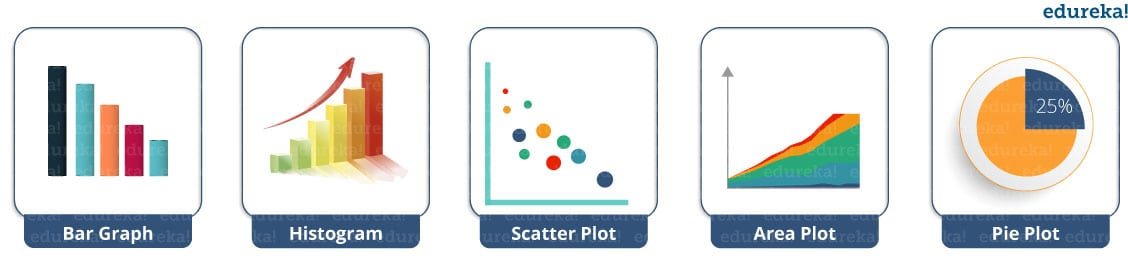I will demonstrate each one of them in detail.

But before that, let me show you very basic codes in python matplotlib in order to generate a simple graph.

``````from matplotlib import pyplot as plt

#Plotting to our canvas

plt.plot([1,2,3],[4,5,1])

#Showing what we plotted

plt.show()``````

Output –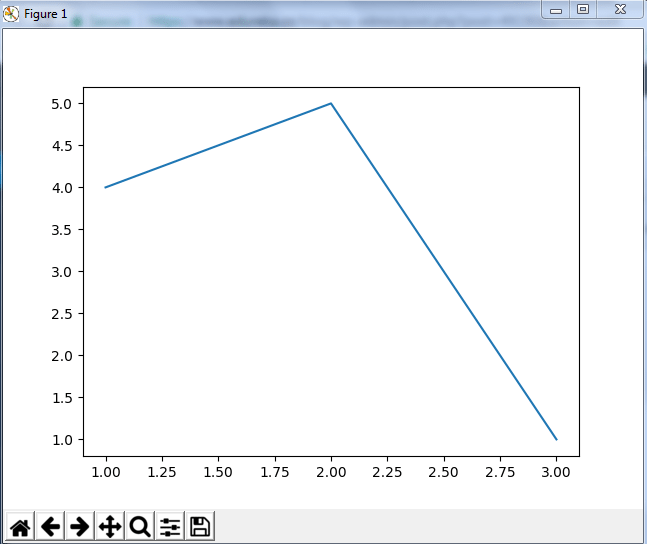So, with three lines of code, you can generate a basic graph using python matplotlib. Simple, isn’t it?
Let us see how can we add title, labels to our graph created by python matplotlib library to bring in more meaning to it. Consider the below example:

``````from matplotlib import pyplot as plt

x = [5,2,7]
y = [2,16,4]
plt.plot(x,y)
plt.title('Info')
plt.ylabel('Y axis')
plt.xlabel('X axis')
plt.show()``````

Output –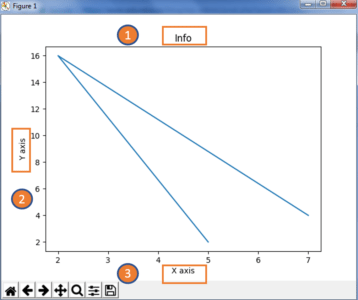You can even try many styling techniques to create a better graph. What if you want to change the width or color of a particular line or what if you want to have some grid lines, there you need styling! So, let me show you how to add style to a graph using python matplotlib. First, you need to import the style package from python matplotlib library and then use styling functions as shown in below code:

``````from matplotlib import pyplot as plt
from matplotlib import style

style.use('ggplot')
x = [5,8,10]
y = [12,16,6]
x2 = [6,9,11]
y2 = [6,15,7]
plt.plot(x,y,'g',label='line one', linewidth=5)
plt.plot(x2,y2,'c',label='line two',linewidth=5)
plt.title('Epic Info')
plt.ylabel('Y axis')
plt.xlabel('X axis')
plt.legend()
plt.grid(True,color='k')
plt.show()``````

Output –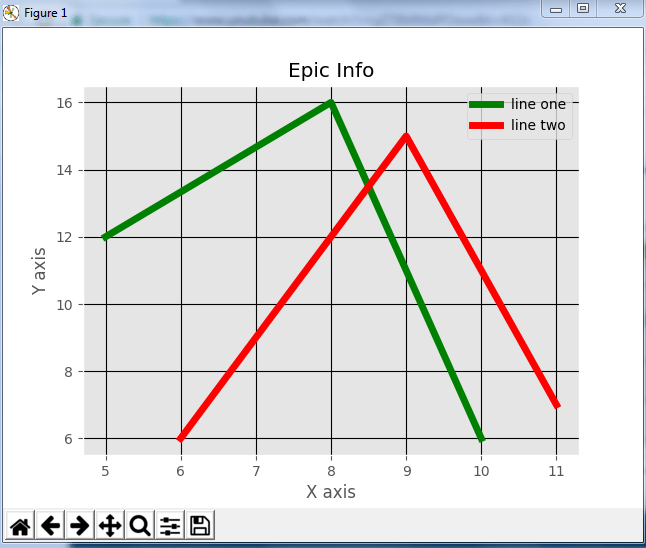Next in this python matplotlib blog, we will understand different kinds of plots. Let’s start with bar graph!

### Python Matplotlib: Bar Graph

First, let us understand why do we need a bar graph. A bar graph uses bars to compare data among different categories. It is well suited when you want to measure the changes over a period of time. It can be represented horizontally or vertically. Also, the important thing to keep in mind is that longer the bar, greater is the value. Now, let us practically implement it using python matplotlib.

``````from matplotlib import pyplot as plt

plt.bar([0.25,1.25,2.25,3.25,4.25],[50,40,70,80,20],
label="BMW",width=.5)
plt.bar([.75,1.75,2.75,3.75,4.75],[80,20,20,50,60],
label="Audi", color='r',width=.5)
plt.legend()
plt.xlabel('Days')
plt.ylabel('Distance (kms)')
plt.title('Information')
plt.show()``````

Output –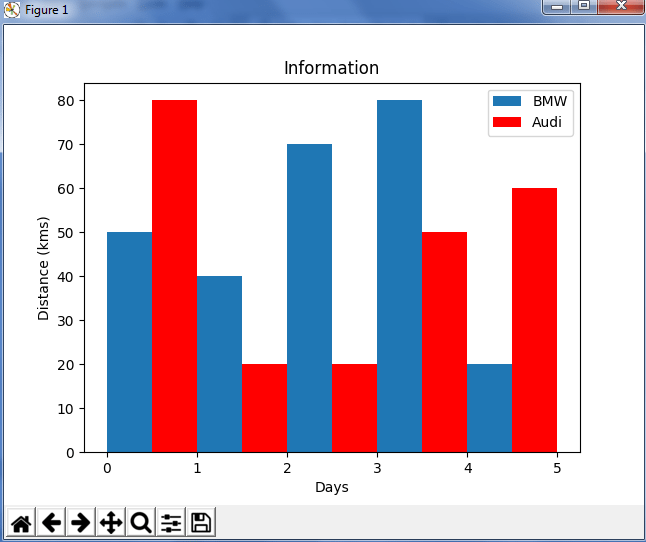In the above plot, I have displayed the comparison between the distance covered by two cars BMW and Audi over a period of 5 days. Next, let us move on to another kind of plot using python matplotlib – Histogram.

### Python Matplotlib – Histogram

Let me first tell you the difference between a bar graph and a histogram. Histograms are used to show a distribution whereas a bar chart is used to compare different entities. Histograms are useful when you have arrays or a very long list. Let’s consider an example where I have to plot the age of population with respect to bin. Now, bin refers to the range of values that are divided into series of intervals. Bins are usually created of the same size. In the below code, I have created the bins in the interval of 10 which means the first bin contains elements from 0 to 9, then 10 to 19 and so on.

``````import matplotlib.pyplot as plt
population_age = [22,55,62,45,21,22,34,42,42,4,2,102,95,85,55,110,120,70,65,55,111,115,80,75,65,54,44,43,42,48]
bins = [0,10,20,30,40,50,60,70,80,90,100]
plt.hist(population_age, bins, histtype='bar', rwidth=0.8)
plt.xlabel('age groups')
plt.ylabel('Number of people')
plt.title('Histogram')
plt.show()``````

Output –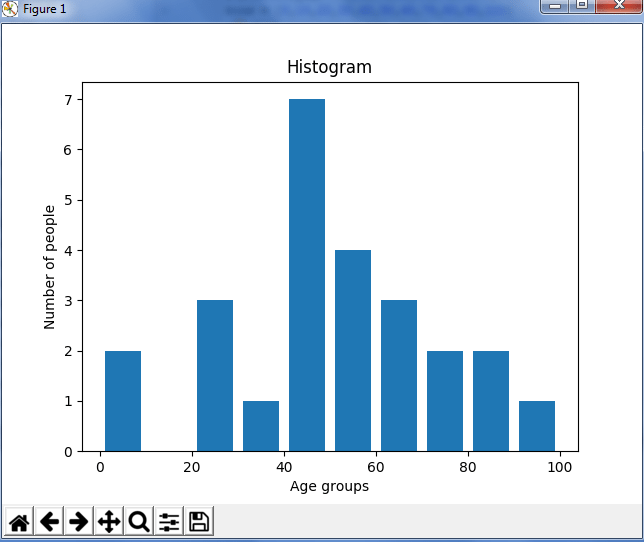As you can see in the above plot, we got age groups with respect to the bins. Our biggest age group is between 40 and 50.

### Python Matplotlib : Scatter Plot

Usually we need scatter plots in order to compare variables, for example, how much one variable is affected by another variable to build a relation out of it. The data is displayed as a collection of points, each having the value of one variable which determines the position on the horizontal axis and the value of other variable determines the position on the vertical axis.

Consider the below example:

``````import matplotlib.pyplot as plt
x = [1,1.5,2,2.5,3,3.5,3.6]
y = [7.5,8,8.5,9,9.5,10,10.5]

x1=[8,8.5,9,9.5,10,10.5,11]
y1=[3,3.5,3.7,4,4.5,5,5.2]

plt.scatter(x,y, label='high income low saving',color='r')
plt.scatter(x1,y1,label='low income high savings',color='b')
plt.xlabel('saving*100')
plt.ylabel('income*1000')
plt.title('Scatter Plot')
plt.legend()
plt.show()``````

Output –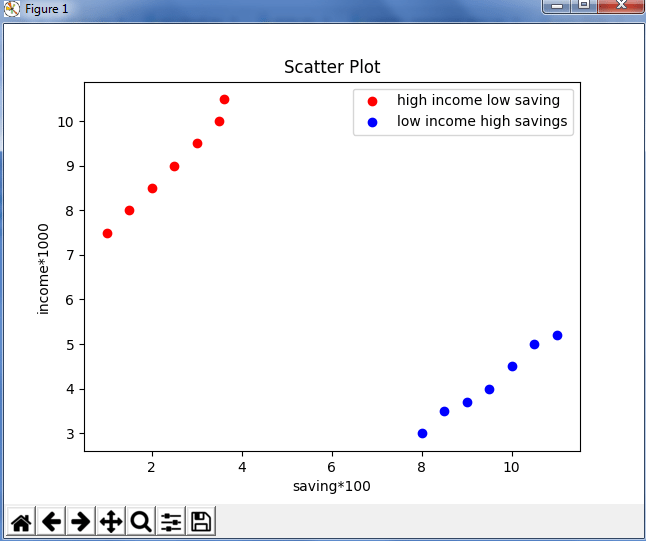As you can see in the above graph, I have plotted two scatter plots based on the inputs specified in the above code. The data is displayed as a collection of points having ‘high income low salary’ and ‘low income high salary’.

Next, let us understand area plot or you can also say Stack plot using python matplotlib.

### Python Matplotlib : Area Plot

Area plots are pretty much similar to the line plot. They are also known as stack plots. These plots can be used to track changes over time for two or more related groups that make up one whole category. For example, let’s compile the work done during a day into categories, say sleeping, eating, working and playing. Consider the below code:

``````import matplotlib.pyplot as plt
days = [1,2,3,4,5]

sleeping =[7,8,6,11,7]
eating = [2,3,4,3,2]
working =[7,8,7,2,2]
playing = [8,5,7,8,13]

plt.plot([],[],color='m', label='Sleeping', linewidth=5)
plt.plot([],[],color='c', label='Eating', linewidth=5)
plt.plot([],[],color='r', label='Working', linewidth=5)
plt.plot([],[],color='k', label='Playing', linewidth=5)

plt.stackplot(days, sleeping,eating,working,playing, colors=['m','c','r','k'])

plt.xlabel('x')
plt.ylabel('y')
plt.title('Stack Plot')
plt.legend()
plt.show()``````

Output –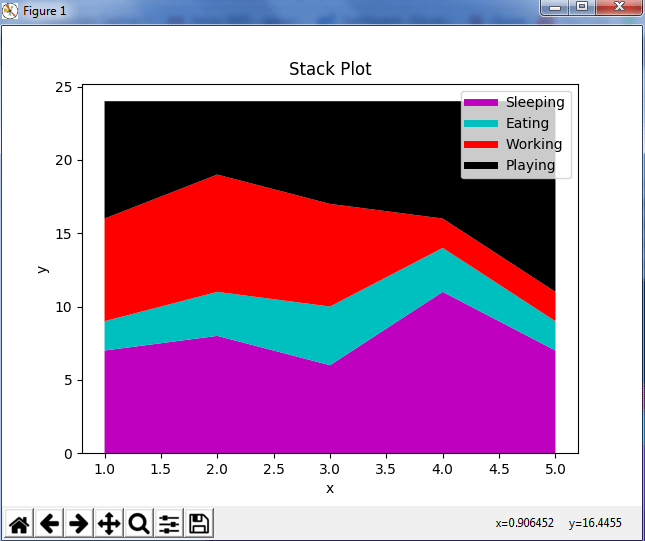As we can see in the above image, we have time spent based on the categories. Therefore, area plot or stack plot is used to show trends over time, among different attributes. Next, let us move to our last yet most frequently used plot – Pie chart.

### Python Matplotlib : Pie Chart

A pie chart refers to a circular graph which is broken down into segments i.e. slices of pie. It is basically used to show the percentage or proportional data where each slice of pie represents a category. Let’s have a look at the below example:

``````import matplotlib.pyplot as plt

days = [1,2,3,4,5]

sleeping =[7,8,6,11,7]
eating = [2,3,4,3,2]
working =[7,8,7,2,2]
playing = [8,5,7,8,13]
slices = [7,2,2,13]
activities = ['sleeping','eating','working','playing']
cols = ['c','m','r','b']

plt.pie(slices,
labels=activities,
colors=cols,
startangle=90,
explode=(0,0.1,0,0),
autopct='%1.1f%%')

plt.title('Pie Plot')
plt.show()``````

Output –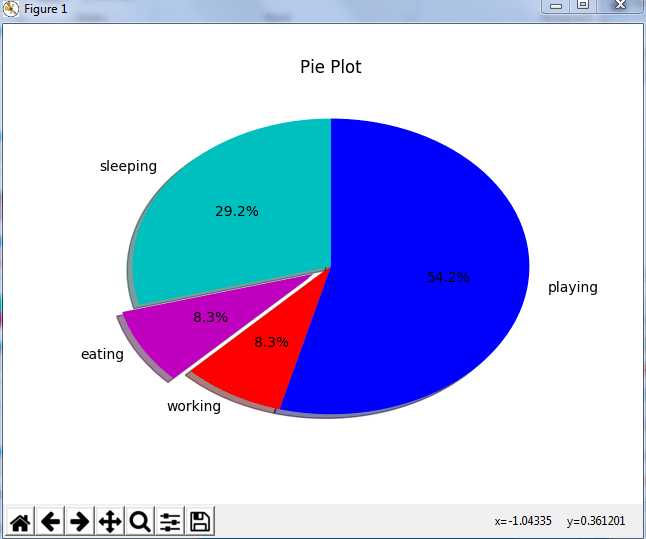In the above pie chart, I have divided the circle into 4 sectors or slices which represents the respective category (playing, sleeping, eating and working) along with the percentage they hold. Now, if you have noticed these slices adds up to 24 hrs, but the calculation of pie slices is done automatically for you. In this way, pie charts are really useful as you don’t have to be the one who calculates the percentage or the slice of the pie.

Next in python matplotlib, let’s understand how to work with multiple plots.

## Python Matplotlib : Working With Multiple Plots

I have discussed about multiple types of plots in python matplotlib such as bar plot, scatter plot, pie plot, area plot etc. Now, let me show you how to handle multiple plots. For this, I have to import numpy module which I discussed in my previous blog on Python Numpy. Let me implement it practically, consider the below example.

``````import numpy as np
import matplotlib.pyplot as plt

def f(t):
return np.exp(-t) * np.cos(2*np.pi*t)
t1 = np.arange(0.0, 5.0, 0.1)
t2 = np.arange(0.0, 5.0, 0.02)
plt.subplot(221)
plt.plot(t1, f(t1), 'bo', t2, f(t2))
plt.subplot(222)
plt.plot(t2, np.cos(2*np.pi*t2))
plt.show()``````

Output -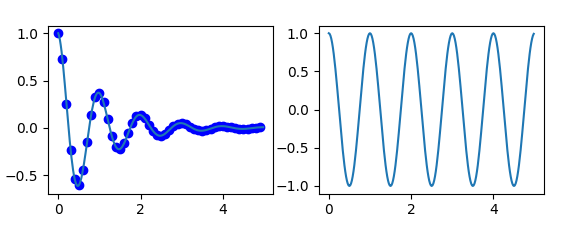The code is pretty much similar to the previous examples that you have seen but there is one new concept here i.e. subplot. The subplot() command specifies numrow, numcol, fignum which ranges from 1 to numrows*numcols. The commas in this command are optional if numrows*numcols<10. So subplot (221) is identical to subplot (2,2,1). Therefore, subplots helps us to plot multiple graphs in which you can define it by aligning vertically or horizontally. In the above example, I have aligned it horizontally.

Apart from these, python matplotlib has some disadvantages. Some of them are listed below:

• They are heavily reliant on other packages, such as NumPy.
• It only works for python, so it is hard or impossible to be used in languages other than python. (But it can be used from Julia via PyPlot package).

We have come to an end of this python matplotlib tutorial. I have covered all the basics of matplotlib, so you can start practicing now. I hope you guys are clear about each and every aspect that I have discussed above. After this python matplotlib blog, I will be coming up with more blogs on python class, scikit learn and array. Stay tuned!

Got a question for us? Please mention it in the comments section of this “Python Matplotlib” blog  and we will get back to you as soon as possible.

To get in-depth knowledge on python along with its various applications, you can enroll for live Python online training by Edureka with 24/7 support and lifetime access.

Python Matplotlib Tutorial | Matplotlib Tutorial | Python Tutorial | Python Training | Edureka

This Edureka Python Matplotlib tutorial explains what is data visualization and how to perform data visualization using Matplotlib.

Next, let us move forward in this blog and explore different types of plots available in python matplotlib.

Original article source at: https://www.edureka.co/

## Buddha Community1619510796

## Lambda, Map, Filter functions in python

Welcome to my Blog, In this article, we will learn python lambda function, Map function, and filter function.

Lambda function in python: Lambda is a one line anonymous function and lambda takes any number of arguments but can only have one expression and python lambda syntax is

Syntax: x = lambda arguments : expression

Now i will show you some python lambda function examples:

#python #anonymous function python #filter function in python #lambda #lambda python 3 #map python #python filter #python filter lambda #python lambda #python lambda examples #python map1626775355

## Why use Python for Software Development

No programming language is pretty much as diverse as Python. It enables building cutting edge applications effortlessly. Developers are as yet investigating the full capability of end-to-end Python development services in various areas.

By areas, we mean FinTech, HealthTech, InsureTech, Cybersecurity, and that's just the beginning. These are New Economy areas, and Python has the ability to serve every one of them. The vast majority of them require massive computational abilities. Python's code is dynamic and powerful - equipped for taking care of the heavy traffic and substantial algorithmic capacities.

Programming advancement is multidimensional today. Endeavor programming requires an intelligent application with AI and ML capacities. Shopper based applications require information examination to convey a superior client experience. Netflix, Trello, and Amazon are genuine instances of such applications. Python assists with building them effortlessly.

## 5 Reasons to Utilize Python for Programming Web Apps

Python can do such numerous things that developers can't discover enough reasons to admire it. Python application development isn't restricted to web and enterprise applications. It is exceptionally adaptable and superb for a wide range of uses.

Robust frameworks

Python is known for its tools and frameworks. There's a structure for everything. Django is helpful for building web applications, venture applications, logical applications, and mathematical processing. Flask is another web improvement framework with no conditions.

Web2Py, CherryPy, and Falcon offer incredible capabilities to customize Python development services. A large portion of them are open-source frameworks that allow quick turn of events.

Python has an improved sentence structure - one that is like the English language. New engineers for Python can undoubtedly understand where they stand in the development process. The simplicity of composing allows quick application building.

The motivation behind building Python, as said by its maker Guido Van Rossum, was to empower even beginner engineers to comprehend the programming language. The simple coding likewise permits developers to roll out speedy improvements without getting confused by pointless subtleties.

Utilized by the best

Alright - Python isn't simply one more programming language. It should have something, which is the reason the business giants use it. Furthermore, that too for different purposes. Developers at Google use Python to assemble framework organization systems, parallel information pusher, code audit, testing and QA, and substantially more. Netflix utilizes Python web development services for its recommendation algorithm and media player.

Massive community support

Python has a steadily developing community that offers enormous help. From amateurs to specialists, there's everybody. There are a lot of instructional exercises, documentation, and guides accessible for Python web development solutions.

Today, numerous universities start with Python, adding to the quantity of individuals in the community. Frequently, Python designers team up on various tasks and help each other with algorithmic, utilitarian, and application critical thinking.

Progressive applications

Python is the greatest supporter of data science, Machine Learning, and Artificial Intelligence at any enterprise software development company. Its utilization cases in cutting edge applications are the most compelling motivation for its prosperity. Python is the second most well known tool after R for data analytics.

The simplicity of getting sorted out, overseeing, and visualizing information through unique libraries makes it ideal for data based applications. TensorFlow for neural networks and OpenCV for computer vision are two of Python's most well known use cases for Machine learning applications.

### Summary

Thinking about the advances in programming and innovation, Python is a YES for an assorted scope of utilizations. Game development, web application development services, GUI advancement, ML and AI improvement, Enterprise and customer applications - every one of them uses Python to its full potential.

The disadvantages of Python web improvement arrangements are regularly disregarded by developers and organizations because of the advantages it gives. They focus on quality over speed and performance over blunders. That is the reason it's a good idea to utilize Python for building the applications of the future.

#python development services #python development company #python app development #python development #python in web development #python software development1619571780

## Top 20 Most Useful Python Modules or Packages

Welcome to my blog, In this article, we will learn the top 20 most useful python modules or packages and these modules every Python developer should know.

Hello everybody and welcome back so in this article I’m going to be sharing with you 20 Python modules you need to know. Now I’ve split these python modules into four different categories to make little bit easier for us and the categories are:

1. Web Development
2. Data Science
3. Machine Learning
4. AI and graphical user interfaces.

Near the end of the article, I also share my personal favorite Python module so make sure you stay tuned to see what that is also make sure to share with me in the comments down below your favorite Python module.

#python #packages or libraries #python 20 modules #python 20 most usefull modules #python intersting modules #top 20 python libraries #top 20 python modules #top 20 python packages1598811780

## Top 7 Python Libraries Used For Hacking

python is one of the most go-for languages among the developers due to the availability of open-source libraries and frameworks. According to a survey reportPython is the top language preferred for Statistical Modelling, and an overwhelming majority of practitioners prefer Python as the language for statistical works.

Python has become a favourite language for hackers these days. The reason is the presence of pre-built tools and libraries, which makes hacking easy. In fact, the language is adequate for ethical hacking as ethical hackers need to develop smaller scripts, and Python fulfils this criterion.

Below here, we listed down the top 7 Python libraries used in hacking.

#### 1| Requests

Stars: 43.3k

**About: **Requests is a simple HTTP library for Python that allows a user to send HTTP/1.1 requests extremely easily. This library helps in building robust HTTP applications and includes intuitive features such as automatic content decompression and decoding, connection timeouts, basic & digits authentication, among others.

Know more here.

#### 2| Scapy

Stars: 5.5k

About: Scapy is a powerful Python-based interactive packet manipulation program and library. This library is able to forge or decode packets of a wide number of protocols, send them on the wire, capture them, store or read them using pcap files, match requests, and more. It allows the construction of tools that can easily scan or attack networks. It is designed to allow fast packet prototyping by using default values that work. It can also perform tasks such as sending invalid frames, injecting your own 802.11 frames, combining techniques, such as VLAN hopping with ARP cache poisoning, VOIP decoding on WEP encrypted channel, etc., which most other tools cannot.

Know more here.

#### 3| IMpacket

**Stars: **5.3k

**About: **IMpacket is a library that includes a collection of Python classes for working with network protocols. It is focused on providing low-level programmatic access to network packets. It allows Python developers to craft and decode network packets in a simple and consistent manner. The library provides a set of tools as examples of what can be done within the context of this library.

Know more here.

#### 4| Cryptography

**Stars: **3.5k

**About: **Cryptography is a package which provides cryptographic recipes and primitives to Python developers. It includes both high-level recipes and low-level interfaces to common cryptographic algorithms such as symmetric ciphers, message digests and key derivation functions. This library is broadly divided into two levels. One is with safe cryptographic recipes that require little to no configuration choices. The other level is low-level cryptographic primitives, which are often dangerous and can be used incorrectly.

Know more here.

#developers corner #hacking tools #libraries for hacking #python #python libraries #python libraries used for hacking #python tools1625100480

## 4 Cool Python Libraries That You Should Know About

### Discover useful Python libraries that you should try out in your next project

Some of my most popular blogs are about Python libraries. I believe that they are so popular because Python libraries have the power to save us a lot of time and headaches. The problem is that most people focus on those most popular libraries but forget that multiple less-known Python libraries are just as good as their most famous cousins.

Finding new Python libraries can also be problematic. Sometimes we read about these great libraries, and when we try them, they don’t work as we expected. If this has ever happened to you, fear no more. I got your back!

In this blog, I will show you four Python libraries and why you should try them. Let’s get started.

#python #coding #programming #cool python libraries #python libraries #4 cool python libraries